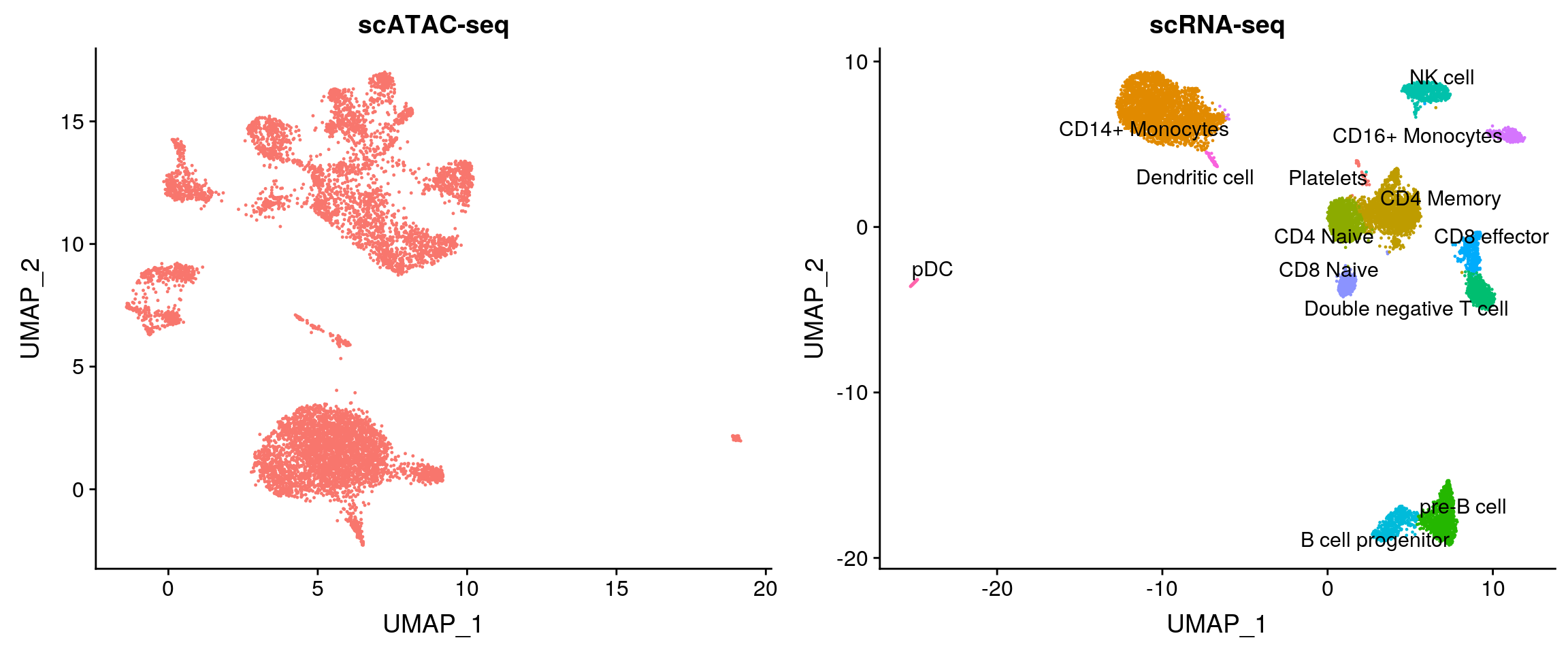In this vignette, we demonstrate our new data transfer method in the context of scATAC-seq to

• Classify cells measured with scATAC-seq based on clustering results from scRNA-seq
• Co-embed scATAC-seq and scRNA-seq data

For this example we’ll be working with the 10X PBMC datasets that contain ~10K cells for both scRNA-seq and scATAC-seq. You can download those here: scATAC-seq, scATAC-seq metadata, scRNA-seq

Overall our integration procedure consists of the following steps:

1. Estimate RNA-seq levels from ATAC-seq (quantify gene expression ‘activity’ from ATAC-seq reads)
2. Learn the internal structure of the ATAC-seq data on its own (accomplished using LSI)
3. Identify ‘anchors’ between the ATAC-seq and RNA-seq datasets
4. Transfer data between datasets (either transfer labels for classification, or impute RNA levels in the ATAC-seq data to enable co-embedding)

# Gene activity quantification

First, we load in the provided peak matrix and collapse the peak matrix to a “gene activity matrix”. Here, we make the simplifying assumption that a gene’s activity can be quantified by simply summing all counts within the gene body + 2kb upstream but our method is compatible with any method that returns a gene by cell matrix (e.g. Cicero).

``````library(Seurat)
library(ggplot2)
# create a gene activity matrix from the peak matrix and GTF, using chromosomes 1:22, X, and Y.
# Peaks that fall within gene bodies, or 2kb upstream of a gene, are considered
activity.matrix <- CreateGeneActivityMatrix(peak.matrix = peaks, annotation.file = "../data/Homo_sapiens.GRCh37.82.gtf",
seq.levels = c(1:22, "X", "Y"), upstream = 2000, verbose = TRUE)``````

# Object setup

Next, we’ll set up the `Seurat` object and store both the original peak counts in the “ATAC” `Assay` and the gene activity matrix in the “RNA” `Assay`. As a QC step, we also filter out all cells here with fewer than 5K total counts in the scATAC-seq data, though you may need to modify this threshold for your experiment.

``````pbmc.atac <- CreateSeuratObject(counts = peaks, assay = "ATAC", project = "10x_ATAC")
pbmc.atac[["ACTIVITY"]] <- CreateAssayObject(counts = activity.matrix)
meta <- read.table("../data/atac_v1_pbmc_10k_singlecell.csv", sep = ",", header = TRUE, row.names = 1,
stringsAsFactors = FALSE)
meta <- meta[colnames(pbmc.atac), ]
pbmc.atac <- subset(pbmc.atac, subset = nCount_ATAC > 5000)
pbmc.atac\$tech <- "atac"``````

# Data preprocessing

Here, we process the gene activity matrix in order to find anchors between cells in the scATAC-seq dataset and the scRNA-seq dataset.

``````DefaultAssay(pbmc.atac) <- "ACTIVITY"
pbmc.atac <- FindVariableFeatures(pbmc.atac)
pbmc.atac <- NormalizeData(pbmc.atac)
pbmc.atac <- ScaleData(pbmc.atac)``````

We also process the peak matrix. Here we perform latent semantic indexing to reduce the dimensionality of the scATAC-seq data. This procedure learns an ‘internal’ structure for the scRNA-seq data, and is important when determining the appropriate weights for the anchors when transferring information. We utilize Latent Semantic Indexing (LSI) to learn the structure of ATAC-seq data, as proposed in Cusanovich et al, Science 2015 and implemented in the `RunLSI` function. LSI is implemented here by performing computing the term frequency-inverse document frequency (TF-IDF) followed by SVD.

We use all peaks that have at least 100 reads across all cells, and reduce dimensionality to 50. The parameters chosen here are inspired by previous scATAC-seq analyses, and we do not typically change them, but you can do so.

``````DefaultAssay(pbmc.atac) <- "ATAC"
VariableFeatures(pbmc.atac) <- names(which(Matrix::rowSums(pbmc.atac) > 100))
pbmc.atac <- RunLSI(pbmc.atac, n = 50, scale.max = NULL)
pbmc.atac <- RunUMAP(pbmc.atac, reduction = "lsi", dims = 1:50)``````

We have previously pre-processed and clustered a scRNA-seq dataset using the standard workflow in Seurat, and provide the object here. You can see that we can identify standard clusters of myeloid and lymphoid PBMCs.

``````pbmc.rna <- readRDS("../data/pbmc_10k_v3.rds")
pbmc.rna\$tech <- "rna"``````
``````p1 <- DimPlot(pbmc.atac, reduction = "umap") + NoLegend() + ggtitle("scATAC-seq")
p2 <- DimPlot(pbmc.rna, group.by = "celltype", label = TRUE, repel = TRUE) + NoLegend() + ggtitle("scRNA-seq")
CombinePlots(plots = list(p1, p2))``````Now, we can identify anchors between the scATAC-seq dataset and the scRNA-seq dataset and use these anchors to transfer the celltype labels we learned from the 10K scRNA-seq data to the scATAC-seq cells.

``````transfer.anchors <- FindTransferAnchors(reference = pbmc.rna, query = pbmc.atac, features = VariableFeatures(object = pbmc.rna),
reference.assay = "RNA", query.assay = "ACTIVITY", reduction = "cca")``````

To transfer the cluster ids, we provide a vector of previously annotated cell type labels for the RNA to the `refdata` parameter. The output will contain a matrix with predictions and confidence scores for each ATAC-seq cell.

``````celltype.predictions <- TransferData(anchorset = transfer.anchors, refdata = pbmc.rna\$celltype,
weight.reduction = pbmc.atac[["lsi"]])
In `FindTransferAnchors`, we typically project the PCA structure from the reference onto the query when transferring between scRNA-seq datasets. However, when transferring across modalities we find that CCA better captures the shared feature correlation structure and therefore set `reduction = 'cca'` here. Additionally, by default in `TransferData` we use the same projected PCA structure to compute the weights of the local neighborhood of anchors that influence each cell’s prediction. In the case of scRNA-seq to scATA-seq transfer, we use the low dimensional space learned by computing an LSI on the ATAC-seq data to compute these weights as this better captures the “internal” structure of the ATAC-seq data.
``````hist(pbmc.atac\$prediction.score.max)